## P(x) = -5x 2 +125x + 37500 is the total profit function of a company, where x is the production of the company.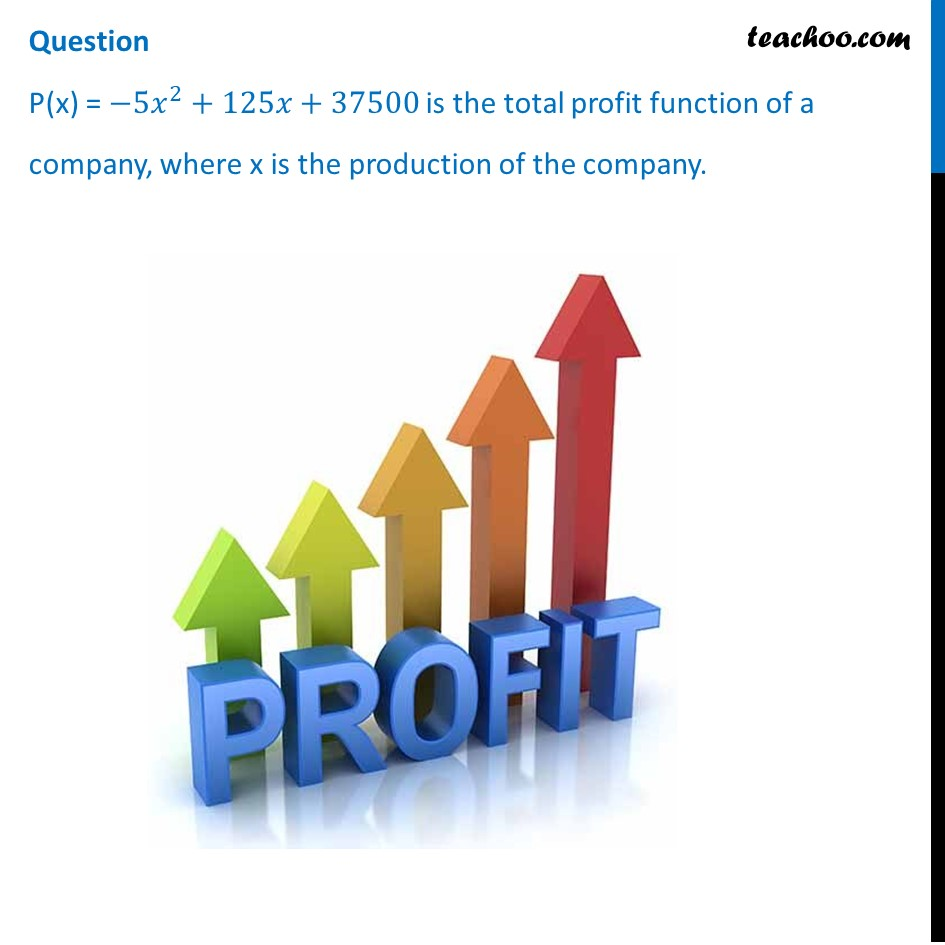## (d) –37500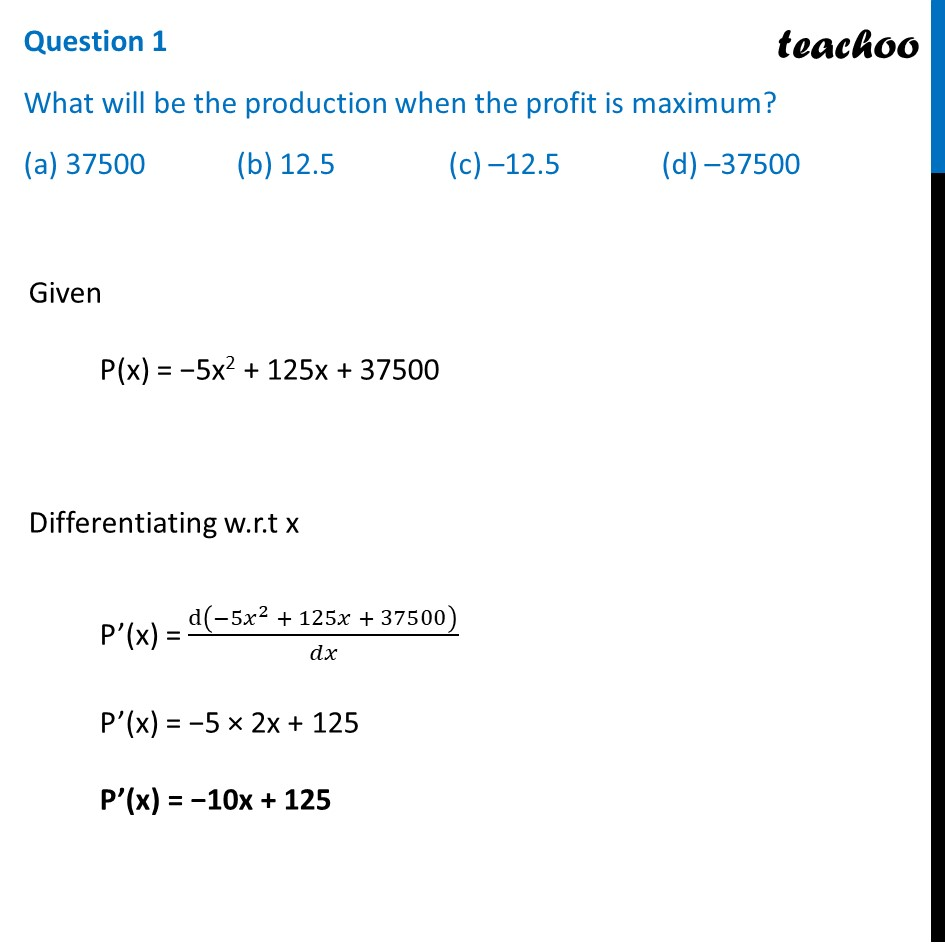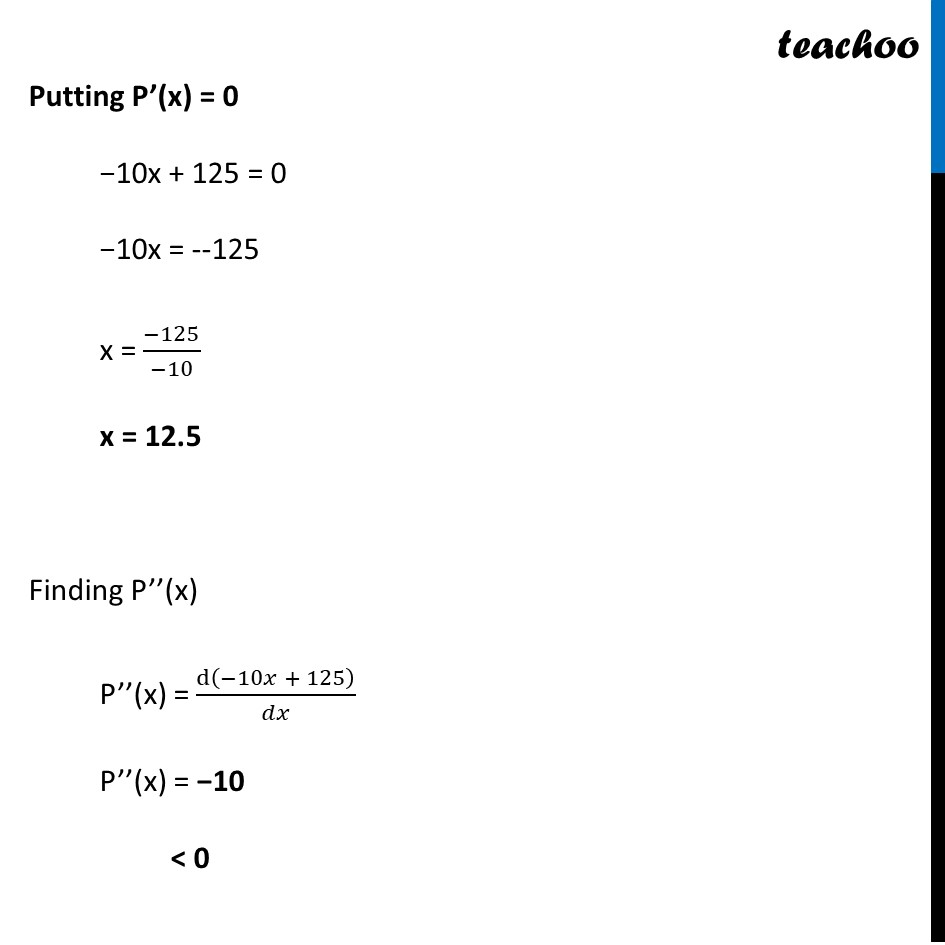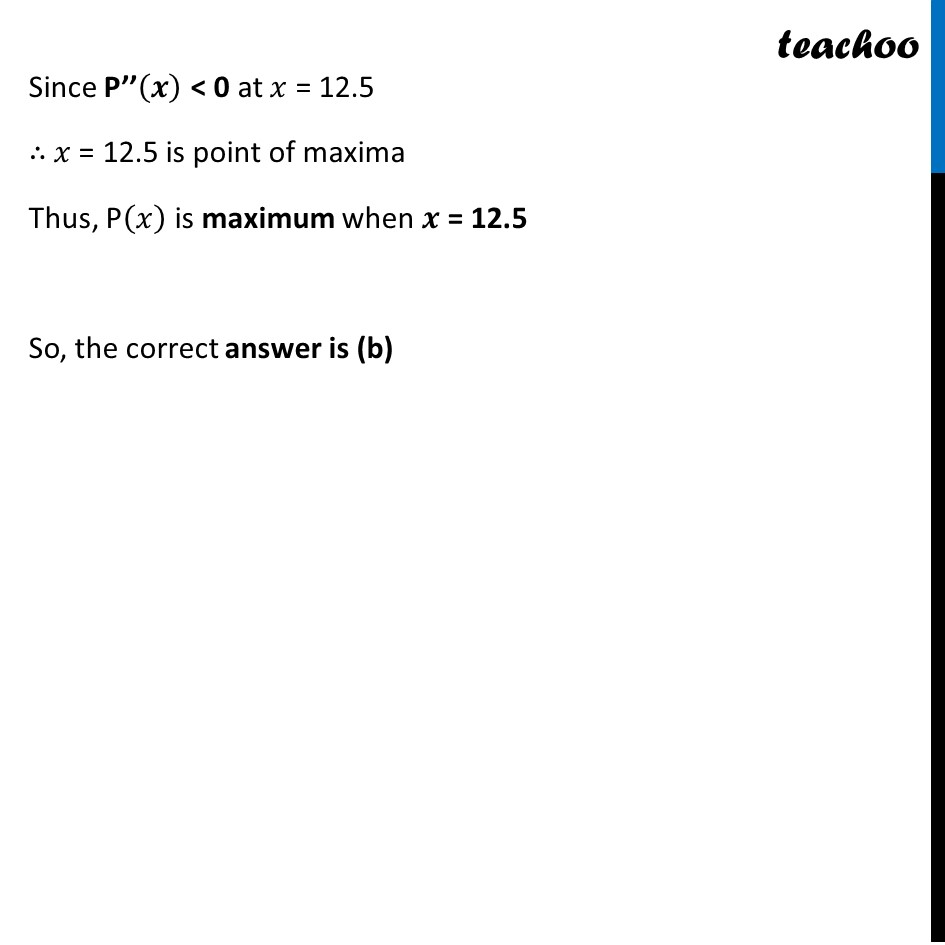## (d) None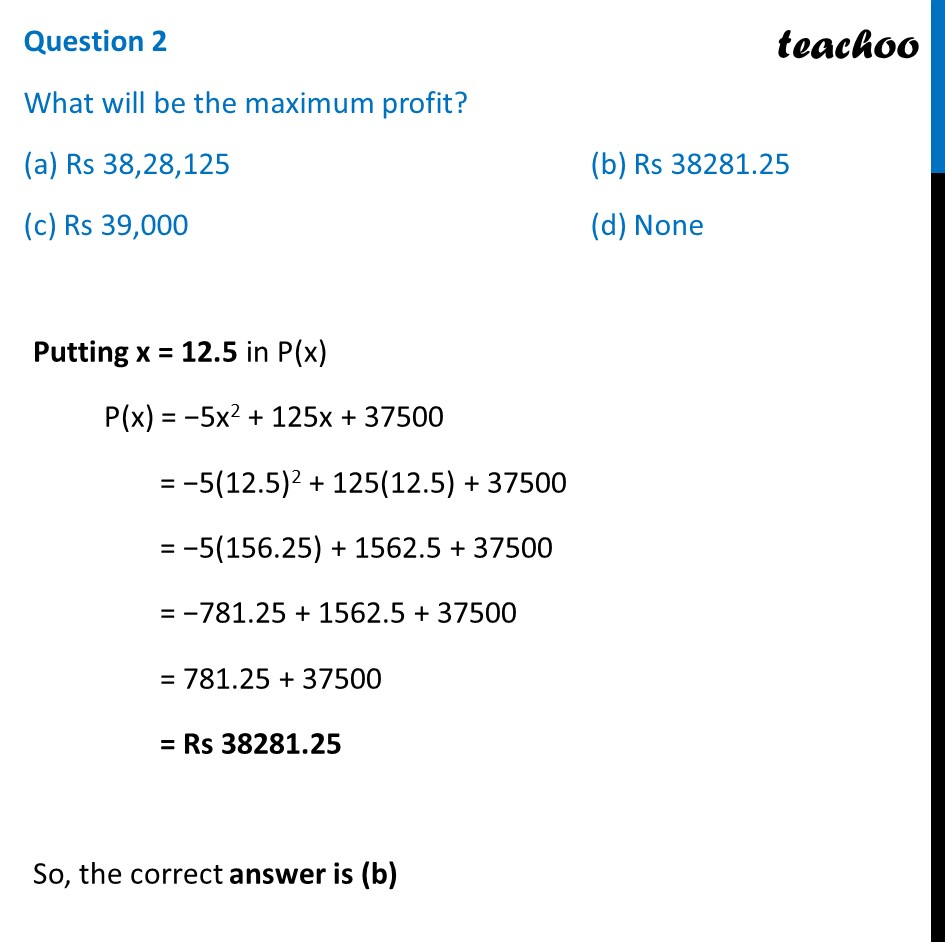## (d) (0, 12.5)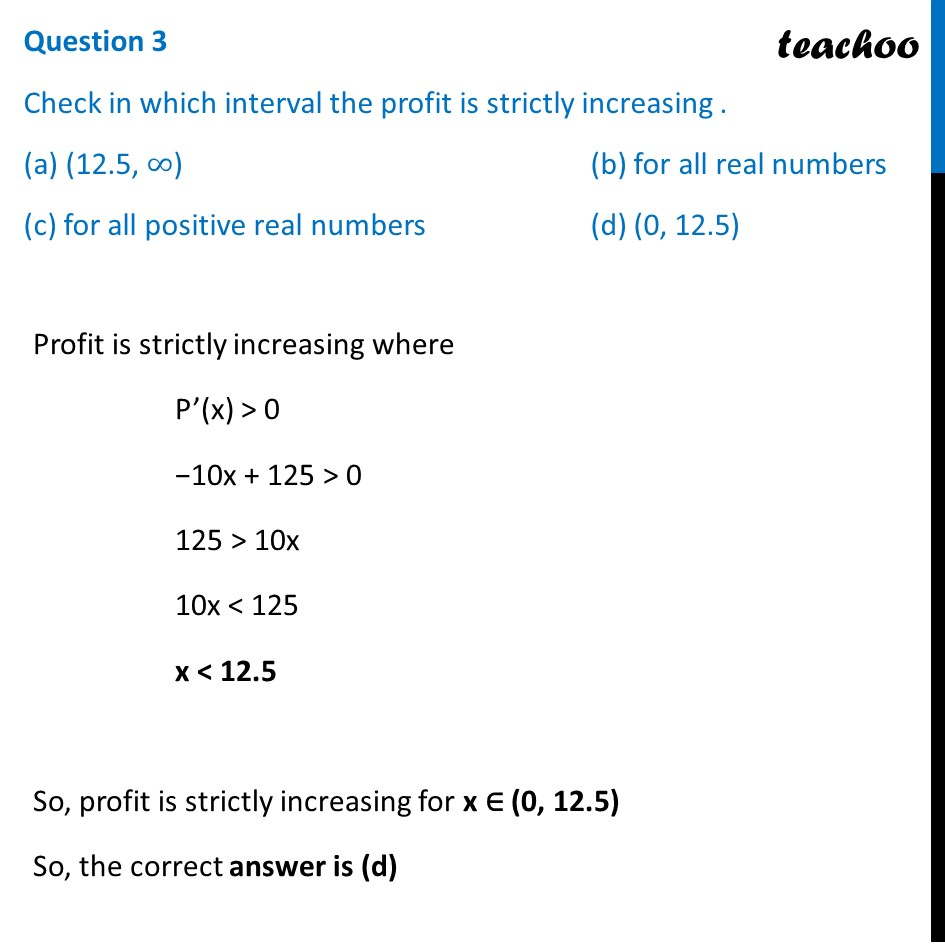## (d) None## (d) data is not sufficient to find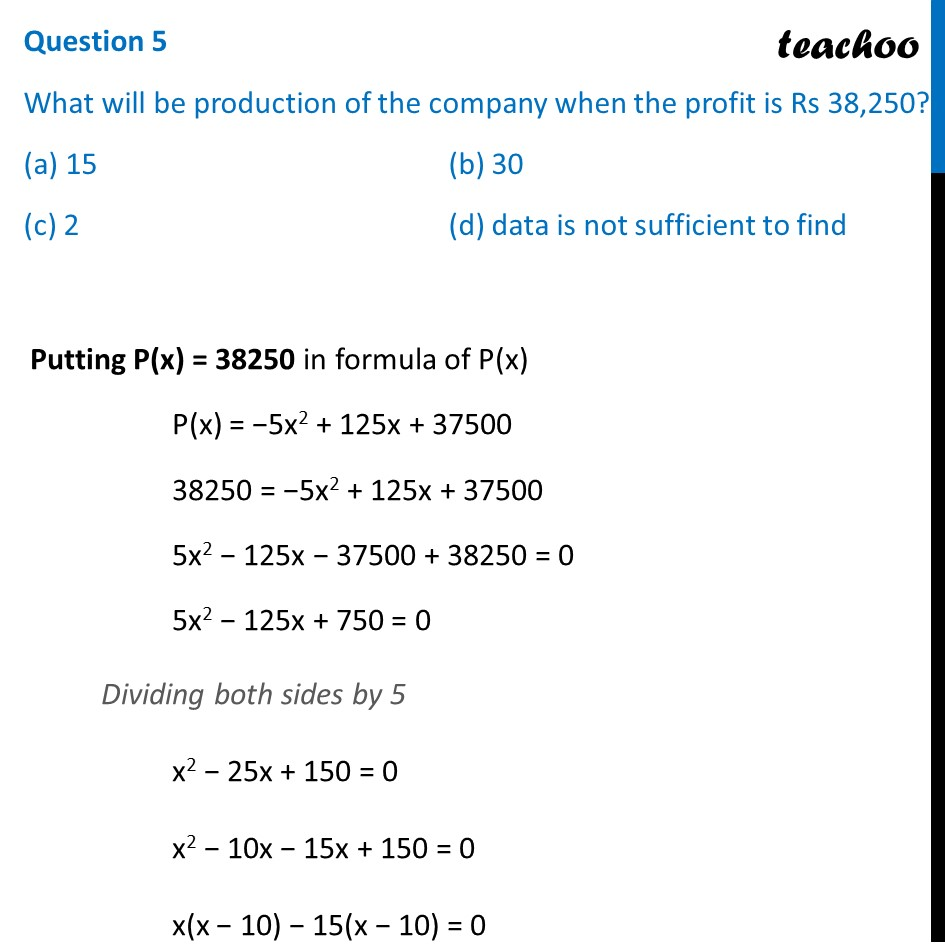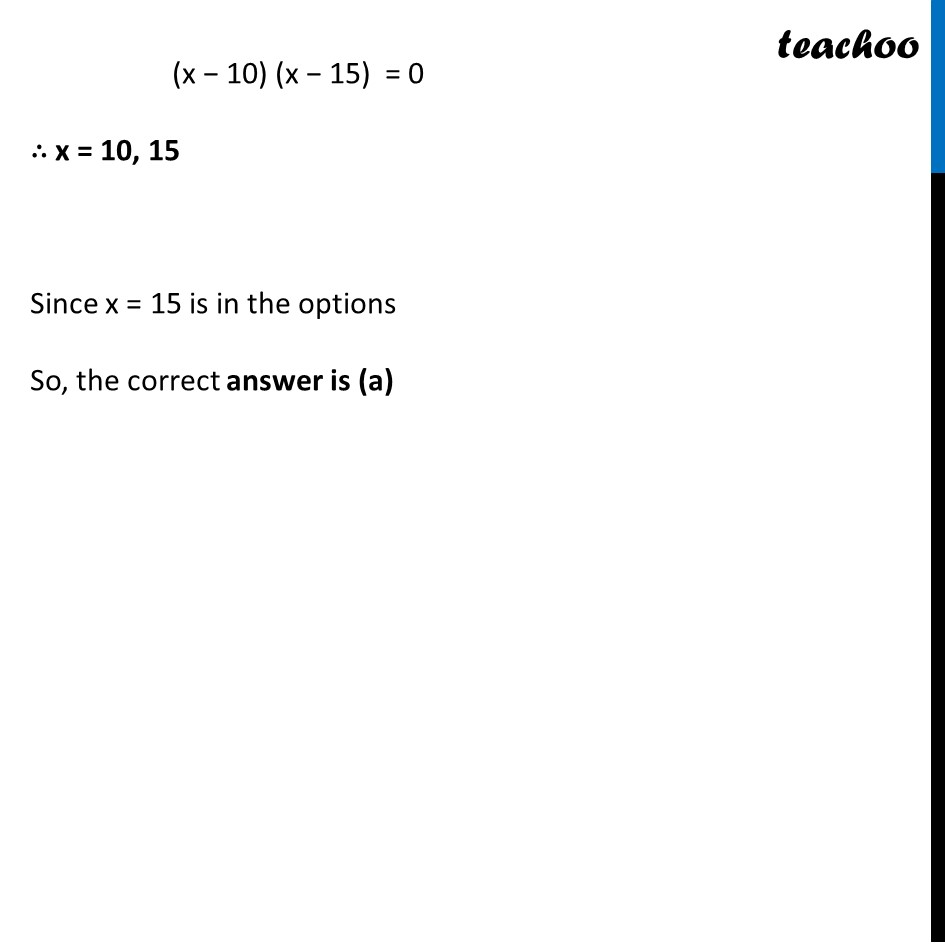1. Chapter 6 Class 12 Application of Derivatives (Term 1)
2. Serial order wise
3. Case Based Questions (MCQ)

Transcript

Question P(x) = 〖−5𝑥〗^2+125𝑥+37500 is the total profit function of a company, where x is the production of the company. Question 1 What will be the production when the profit is maximum? (a) 37500 (b) 12.5 (c) –12.5 (d) –37500 Given P(x) = −5x2 + 125x + 37500 Differentiating w.r.t x P’(x) = d(〖−5𝑥〗^2 + 125𝑥 + 37500)/𝑑𝑥 P’(x) = −5 × 2x + 125 P’(x) = −10x + 125 Putting P’(x) = 0 −10x + 125 = 0 −10x = --125 x = (−125)/(−10) x = 12.5 Finding P’’(x) P’’(x) = d(−10𝑥 + 125)/𝑑𝑥 P’’(x) = −10 < 0 Since P’’(𝒙) < 0 at 𝑥 = 12.5 ∴ 𝑥 = 12.5 is point of maxima Thus, P(𝑥) is maximum when 𝒙 = 12.5 So, the correct answer is (b) Question 2 What will be the maximum profit? (a) Rs 38,28,125 (b) Rs 38281.25 (c) Rs 39,000 (d) None Putting x = 12.5 in P(x) P(x) = −5x2 + 125x + 37500 = −5(12.5)2 + 125(12.5) + 37500 = −5(156.25) + 1562.5 + 37500 = −781.25 + 1562.5 + 37500 = 781.25 + 37500 = Rs 38281.25 So, the correct answer is (b) Question 3 Check in which interval the profit is strictly increasing . (a) (12.5, ∞) (b) for all real numbers (c) for all positive real numbers (d) (0, 12.5) Profit is strictly increasing where P’(x) > 0 −10x + 125 > 0 125 > 10x 10x < 125 x < 12.5 So, profit is strictly increasing for x ∈ (0, 12.5) So, the correct answer is (d) Question 4 When the production is 2 units, what will be the profit of the company? (a) 37,500 (b) 37,730 (c) 37,770 (d) None Putting x = 2 in P(x) P(x) = −5x2 + 125x + 37500 = −5(2)2 + 125(2) + 37500 = −5(4) + 250 + 37500 = −20 + 250 + 37500 = 230 + 37500 = Rs 37730 So, the correct answer is (b) Question 5 What will be production of the company when the profit is Rs 38,250? (a) 15 (b) 30 (c) 2 (d) data is not sufficient to find Putting P(x) = 38250 in formula of P(x) P(x) = −5x2 + 125x + 37500 38250 = −5x2 + 125x + 37500 5x2 − 125x − 37500 + 38250 = 0 5x2 − 125x + 750 = 0 Dividing both sides by 5 x2 − 25x + 150 = 0 x2 − 10x − 15x + 150 = 0 x(x − 10) − 15(x − 10) = 0 (x − 10) (x − 15) = 0 ∴ x = 10, 15 Since x = 15 is in the options So, the correct answer is (a)

Case Based Questions (MCQ)